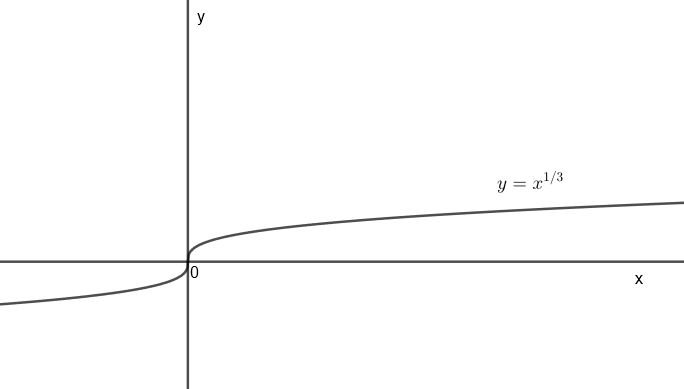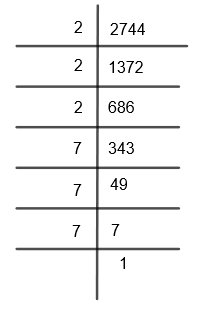Courses
Courses for Kids
Free study material
Free LIVE classes
MoreLIVE
Join Vedantu’s FREE Mastercalss

# Find the cube root of 2744.Verified
268.5k+ views
Hint: First of all let 2744 = a. To find the cube root of 2744, first of all factorize it and then find the cube root that is ${{a}^{\dfrac{1}{3}}}$ or ${{\left( 2744 \right)}^{\dfrac{1}{3}}}$.

Here, we have to find the cube root of 2744.
Before proceeding with this question, we must know what a cube root is.
Cube root of a number is a special value that, when used in a multiplication three times, gives that number. In other words, we can say that the cube root of a number ‘x’ is a number ‘y’ such that $y\times y\times y=x$ or ${{y}^{3}}=x$. Therefore, we get the cube root of x, that is $y={{x}^{\dfrac{1}{3}}}$.
The graph for $y={{x}^{\dfrac{1}{3}}}$ can be drawn asThis plot is symmetric with respect to the origin.
Now, here we have to find the cube root of 2744.
Let us take a = 2744.
Therefore, we get the cube root of 2744 as,
${{a}^{\dfrac{1}{3}}}={{\left( 2744 \right)}^{\dfrac{1}{3}}}....\left( i \right)$
First of all, we will resolve 2744 into its factors as follows. Now, we will use the method of factorization, that is,Therefore, we get $2744=2\times 2\times 2\times 7\times 7\times 7$
We can also write it as, $2744={{2}^{3}}{{.7}^{3}}$.
By putting the value of 2744 in equation (i), we get
${{a}^{\dfrac{1}{3}}}={{\left( {{2}^{3}}{{.7}^{3}} \right)}^{\dfrac{1}{3}}}$
We know that ${{a}^{n}}.{{b}^{n}}={{\left( ab \right)}^{n}}$.
By applying this in the above equation, we get,
${{a}^{\dfrac{1}{3}}}={{\left( {{14}^{3}} \right)}^{\dfrac{1}{3}}}$
We know that ${{\left( {{a}^{n}} \right)}^{m}}={{a}^{nm}}$. By applying this in the above equation, we get,
${{a}^{\dfrac{1}{3}}}={{\left( 14 \right)}^{3\times \dfrac{1}{3}}}$
Therefore, we get ${{a}^{\dfrac{1}{3}}}=14$
Hence, we have found that the cube root of 2744 is 14.

Note: Students should properly factorize the given number and cross-check if they are getting the same number by multiplication of factors or not. Also, after finding the cube root, students can take a cube of that cube root to find if it is giving the correct number or not. Students can cross-check in this way.
As we have found that the cube root of 2744 is 14.
We will find ${{14}^{3}}=14\times 14\times 14$
We get, ${{14}^{3}}=2744$